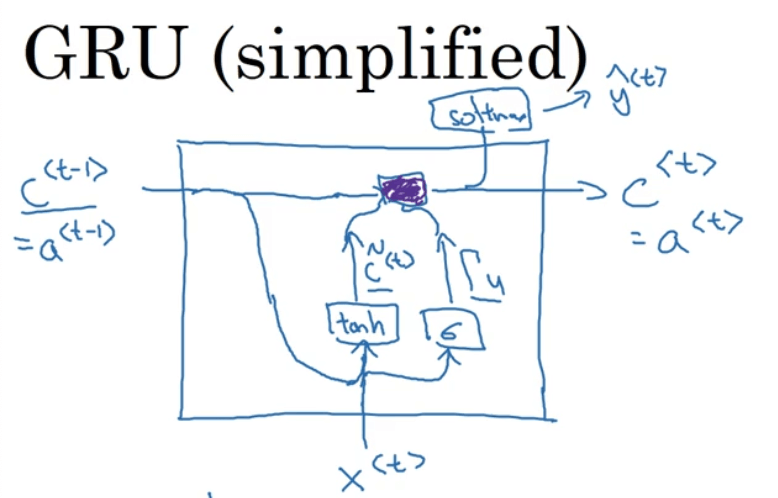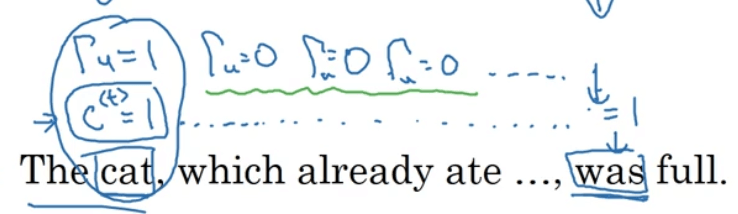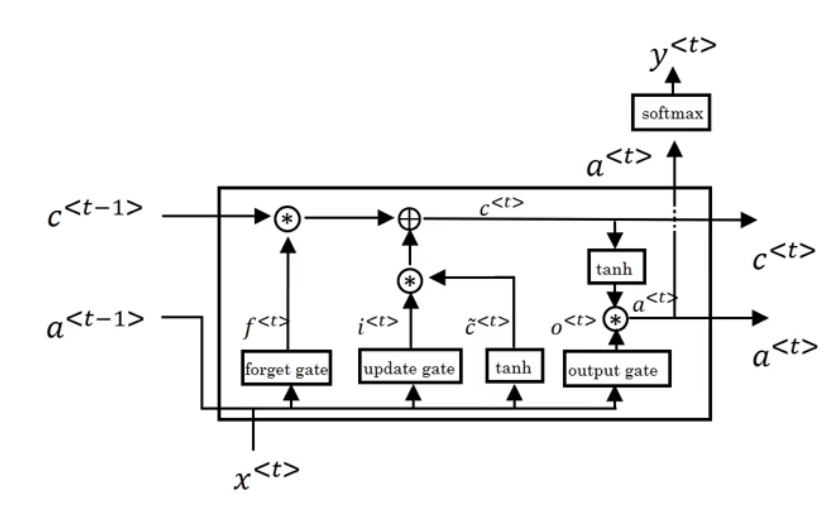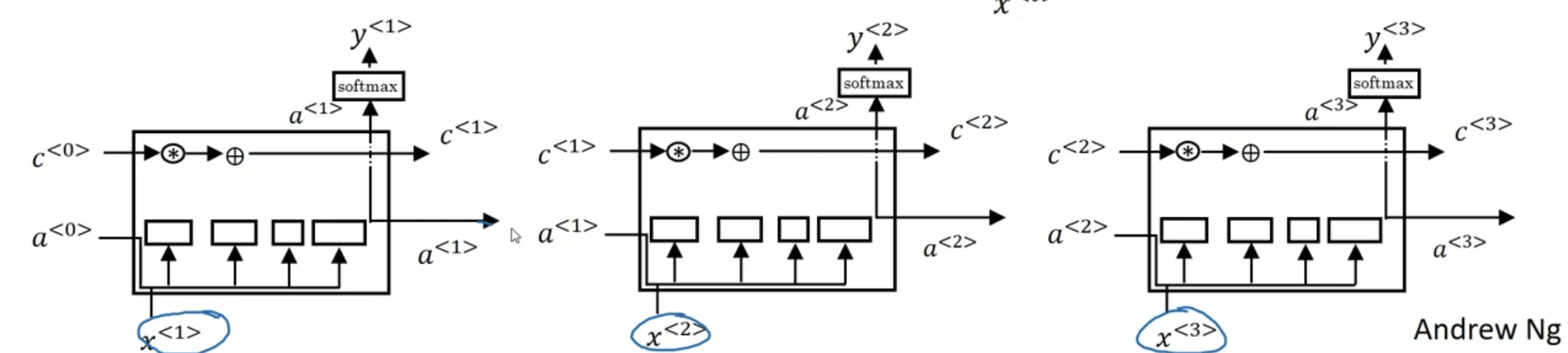# 【深度学习基础】第四十二课：GRU和LSTM

## GRU，LSTM

Posted by x-jeff on December 9, 2020

【深度学习基础】系列博客为学习Coursera上吴恩达深度学习课程所做的课程笔记。

# 1.RNN中的梯度消失问题

【深度学习基础】第四十一课：RNN应用之语言模型中的语言模型为例，假设我们有句子：“The cat , which …… , was full.”，其中“cat”和“was”需要对应起来。如果使用复数“cats”，则对应的需要使用“were”：“The cats , which …… , were full.”。对于英语来说，“which”后面省略的部分可以是任意长度，因此就需要“cat/cats”和“was/were”之间有长期的依赖关系。但是目前为止，我们所介绍的RNN模型都不擅长捕获这种长期依赖效应。而造成此问题的原因就是梯度消失

# 2.GRU

GRU的全称为Gated Recurrent Unit

GRU的介绍主要来自以下两篇文章：

1. Cho K, Van Merriënboer B, Bahdanau D, et al. On the properties of neural machine translation: Encoder-decoder approaches[J]. arXiv preprint arXiv:1409.1259, 2014.

2. Chung J, Gulcehre C, Cho K H, et al. Empirical evaluation of gated recurrent neural networks on sequence modeling[J]. arXiv preprint arXiv:1412.3555, 2014.

GRU的基本结构如下图所示：$\tilde {c}^{<t>}=tanh(W_c [c^{<t-1>},x^{<t>}]+b_c)$ $\Gamma _u=sigmoid(W_u [c^{<t-1>},x^{<t>}]+b_u)$

$c^{<t>}=\Gamma _u * \tilde {c}^{<t>} + (1-\Gamma _u) * c^{<t-1>}$GRU的优点：

• 使用Gate控制memory cell的更新。
• 因为$c^{<t>}$可能会被持续的传递下去，因此解决了梯度消失问题。

$\tilde {c}^{<t>}=tanh(W_c [\Gamma _r * c^{<t-1>},x^{<t>}]+b_c)$ $\Gamma _r=sigmoid(W_r [c^{<t-1>},x^{<t>}]+b_r)$

# 3.LSTM

LSTM的全称为Long Short Term Memory，其比GRU要更为强大和通用。

LSTM出自论文：Hochreiter S, Schmidhuber J. Long short-term memory[J]. Neural computation, 1997, 9(8): 1735-1780.$\tilde c^{<t>}=tanh(W_c [a^{<t-1>},x^{<t>}]+b_c)$ $\Gamma _u=sigmoid(W_u [a^{<t-1>},x^{<t>}]+b_u)$ $\Gamma _f=sigmoid(W_f [a^{<t-1>},x^{<t>}]+b_f)$ $\Gamma _o=sigmoid(W_o [a^{<t-1>},x^{<t>}]+b_o)$ $c^{<t>}=\Gamma _u * \tilde c^{<t>} + \Gamma _f * c^{<t-1>}$ $a^{<t>}=\Gamma _o * tanh( c^{<t>})$